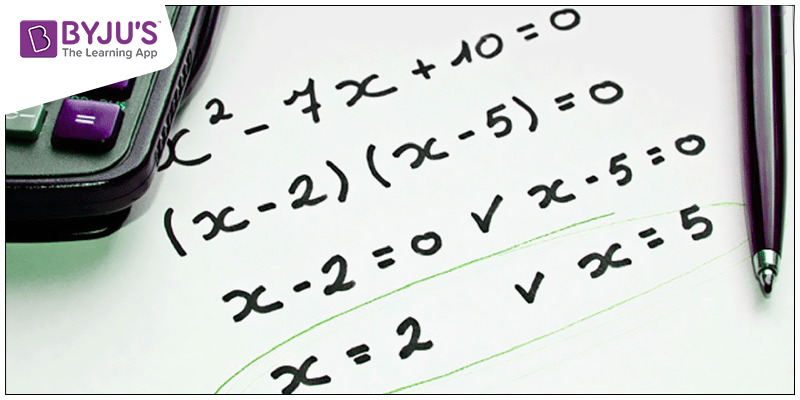# GMAT Algebra Sample QuestionsIn GMAT quant section, typically you’ll find questions from algebra – Linear Equations. These types of questions are usually a part of Data Sufficiency which analyzes your understanding of the different types of solutions.

Here are few sample questions on GMAT Algebra to equip an idea on Quant section –

## Solving Equations With Absolute Values

1) $Solve \; for \; x, \; Where \; |x + 5| – 10 = 0$

Solution – $|x + 5| – 10 = 0$

By adding 10 in both sides –

$|x + 5| = 10$

Without brackets,

$x + 5 = 10$

$x = 5$

Without brackets, multiply the right side by -1 since the expression in the absolute value brackets could be negative due to the effect of the absolute value.

$x + 5 = (10) (-1)$

$x + 5 = -10$

Therefore, x = 5 or x = -15

## Solving an Exponential Equation

2) $Find \; x, \; where \; (8^{2x})^{2} = 64^{x – 2}$

Solution – $As \; 8 = 2^{3} \; and \; 64 = 2^{6}$

$(8^{2x})^{2} = 64^{x – 2}$

$((2^{3})^{2x})^{2} = (2^{6})^{x – 2}$

$2^{3 \times 4x} = 2^{6x – 12}$

$2^{12x} = 2^{6x – 12}$

We can state as further –

$12x = 6x – 12$

$6x = – 12$ (by subtracting 6x from both sides)

Therefore, $x = – 2$

3) $Find \; x, \; when \; 9x – 3 = \frac{2x – 4}{3}$

Solution:

By multiplying 3 on both sides,

$27x – 9 = 2x – 4$

$25x = 5$

Therefore, $x = \frac{1}{5}$

4) $Find \; x, \; if \; x^{2} + 6x + 6 = 0$

Solution:

Using the formula, $x = \frac{-b \pm \sqrt{b^{2} – 4ac}}{2a}$

$x = \frac{-6 \pm \sqrt{6^{2} – 4(1)(6)}}{2(1)}$

$x = \frac{-6 \pm \sqrt{36 – 24}}{2}$

$x = -3 \pm \frac{\sqrt{12}}{2}$

$x = -3 \pm 2\frac{\sqrt{3}}{2}$

$x = -3 \pm \sqrt{3}$

We’ll be glad to help you in your GMAT preparation journey. You can ask for any assistance related to GMAT and MBA from us by calling us at +918884544444. You can write to us at gmat@byjus.com.